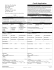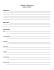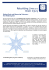# Inverse Square Law

## Transcription

Inverse Square Law
```The Inverse Square Law
In this document we use the inverse square law to show the mathematical relationship between the
distances of stars, their relative brightness, their relative luminosity and their magnitude difference.
Suppose Star A has a luminosity of LA and is at a distance of dA while Star B has a luminosity of LB and is
at a distance of dB. What is the ratio of the brightness of star A (bA) to the brightness of star B (bB)? The
inverse square law tells us that
LA
2
bA d A2 d B2 LA " d B % LA
=
= ×
=\$ ' ×
bB LB d A2 LB \$# d A '& LB
db2
equation 1
So we can see the easiest way to do the math here is to find the ratio of the distance and square that ratio
rather than squaring the distance terms first. If both stars are the same luminosity (LA = LB), then the L
terms cancel and the result tells us how much brighter the nearer star is as show below.
2
bA ! d B \$
=# &
bB #" d A &%
equation 2
Now suppose the two stars are at different distances but appear to have the same brightness as seen from
Earth so that bA = bB. How can we figure the ratio of the luminosities? We begin with equation 1 and set
the left dis of the equation to 1 giving us the expression below which we solve for the ratio of the
luminosities.
2
!d \$ L
1 = ## B && × A
" d A % LB
2
LA ! d A \$
=# &
LB #" d B &%
equation 3
If you think about this expression for a minute you will see that it makes sense. If both stars appear
equally bright as seen from Earth, then the more distant star is the brighter one.
Now to change a brightness ratio bA / bB or a luminosity ratio LA / LB to a magnitude difference we can use
Table 2 in our magnitude handout or the formula uses to calculate the table as given below.
#b &
Δm = 2.512 × log %% A ((
\$ bB '
#L &
ΔM = 2.512 × log %% A ((
\$ LB '
equation 4a
equation 4b
In equation 4a we are calculating the difference in apparent magnitude because we are using the ratio of
apparent brightness but in equation 4b we obtain the difference in absolute magnitudes.
```

### Lab 5 Takehome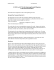### H-R diagram worksheet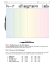### LIFE OF A STAR WORKSHEET (answer key)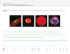### PROBLEM SET #6 AST142 Due in class Tuesday Mar 17, 2015 First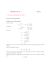### Kimbertal Kennels Star Dog Breeder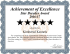### LED Light Cube - 17" Square and 17" tall. 12 lbs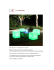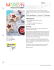### Scanned Document